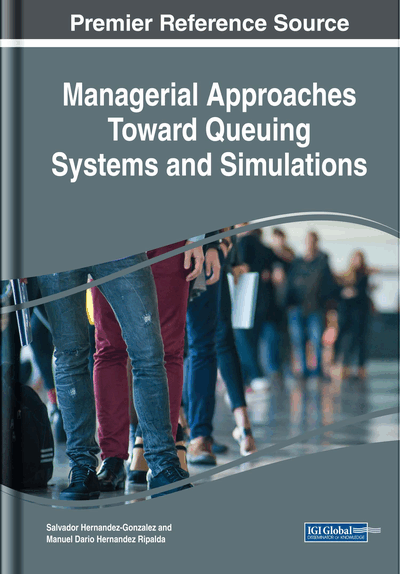# Single-Stage Non-Markovian Systems and Variability

DOI: 10.4018/978-1-5225-5264-2.ch004
Available
\$37.50
No Current Special Offers

## Abstract

The variability of a queueing system is the main theme of Chapter 4; some of the approaches that have been developed to obtain the performance measures are presented. Part of the material is devoted to variability due to equipment failures and their effect on performance measures, as well as in estimating the required capacity. The chapter ends with the topic on propagation of variability in a queue and the respective approximations to calculate the variability at the exit.
Chapter Preview
Top

## Pollacheck-Kintchine Approximation For The Cycle Time

We must remember that, in the M/M/1 case, the evolution of the system is defined by the number of customers present in instant t and, in consequence, the stochastic process {N(t), t > 0} is a Markov chain. For M/G/1 systems, the {N(t), t > 0} process is not a Markov chain as, when there is a customer in the system and a new arrival increases the number of customers in the system, N(t) ≥ 1, the service time that has elapsed (residual time) for the customer that was already there has to be considered, which does not conform with the lack-of-memory property of Markov processes. So, the state diagram does not apply in this case (Stewart, 2009).

Let us assume that demand λ follows a Poisson distribution and that the service discipline is First In, First Out and independent of the service time; let us also assume that for the service time, the only specification is for it to follow a general probability distribution (G) with mean tS and standard deviation σS and for the station to have a single server. Under Kendall’s notation, these types of systems are M/G/1 and M/G/c. The time spent in queue for an M/G/1 system is obtained using the following expression:

(1) where the parameter C2S = varS / (ts)2 is the squared coefficient of variation or simply the variability. We must bear in mind that C2S is a measure of dispersion, while (1) is known as a Pollaczek-Kintchine Formula and, just like the Markov systems, the system has to be stable, in other words, ρ = λtS <1.

The average work in process of the system is:

(2)

Or alternatively:

(3)

Observe that if C2S = 1, then formulas (1) and (3) are the relationships for Markovian systems.

## Complete Chapter List

Search this Book:
Reset